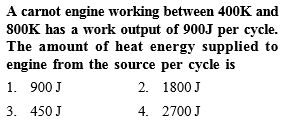You’ve reached the end of your free Videos limit.
#54 | Solved Example: 24
(Physics) > Thermodynamics
Related Practice Questions :Complete Question Bank + Test Series
Complete Question Bank

Difficulty Level:

A carnot engine having an efficiency of$\frac{1}{10}$th of heat engine, is used as a refrigerator. If the work done on the system is 10 J, the amount of energy absorbed from the reservoir at lower temperature is -

(a) 1 J

(b) 90 J

(c) 99 J

(d0 100 J

Complete Question Bank + Test Series
Complete Question Bank

Difficulty Level:

The temperature inside a refrigerator is and the room temperature is . The amount of heat delivered to the room for each joule of electrical energy consumed ideally will be -

(a)$\frac{{t}_{1}}{{t}_{1}-{t}_{2}}$      (b) $\frac{{t}_{1}+273}{{t}_{1}-{t}_{2}}$

(c) $\frac{{t}_{2}+273}{{t}_{1}-{t}_{2}}$    (d) $\frac{{t}_{1}+{t}_{2}}{{t}_{1}+273}$

Complete Question Bank + Test Series
Complete Question Bank

Difficulty Level:

A refrigerator works between 4°C and 30°C. It is required to remove 600 calories of heat every second in order to keep the temperature of the refrigerated space constant. The power required is (Take, 1 cal = 4.2 Joules)

(a)23.65W
(b)236.5W
(c)2365W
(d)2.365W

Complete Question Bank + Test Series
Complete Question Bank

Difficulty Level:

A Carnot engine working between 300 K and 600K has work output of 800 J per cycle. What is amount of heat energy supplied to the engine from source per cycle ?

(1) 1800 J/cycle

(2) 1000 J/cycle

(3) 2000 J/cycle

(4) 1600 J/cycle